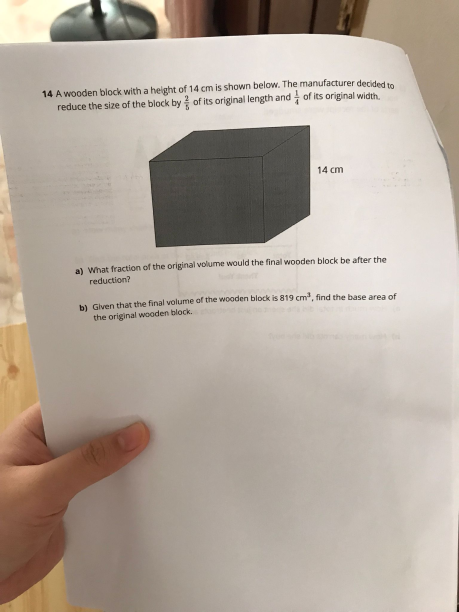# Questionappreciate some assistance to solve the question

(a) Assume the length of the wooden block  is L and the width is W

Volume of block before reduction = 14LW

Length of block after reduction = (3/5) L

Breadth of block after reduction = (3/4)W

Volume of block after reduction = (3/5)L x (3/4) W x 14  = [ (9 x 14) /20 ] LW

Hence fraction final volume of the  block against the original volume

=      [ ( 9 x 14) /20 ] LW

——————–

14 LW

= 9/20

(b)    9u -> 819  => u = 91   Hence 20u = 1820

Let BA = base area of the original block

BA x height = 1820

BA x 14 = 1820

BA = 1820 / 14  = 130

Hence base area of the original block = 130cm2